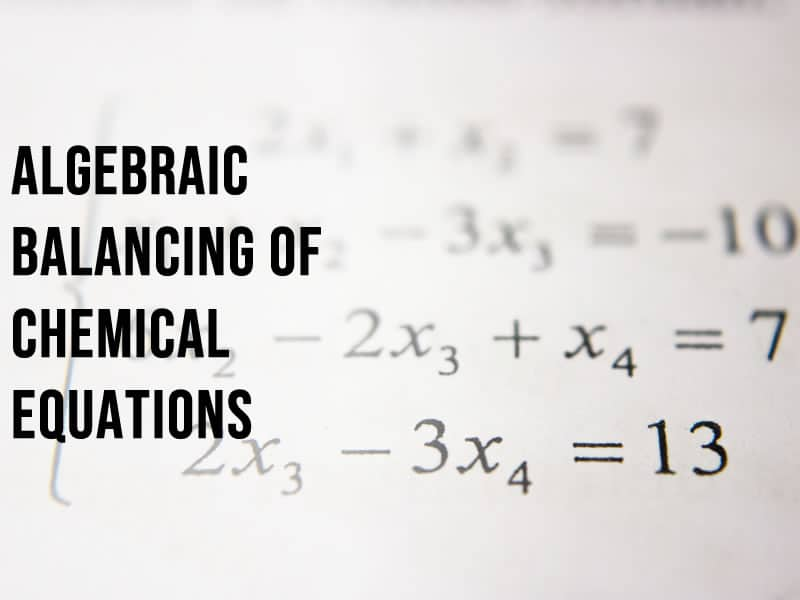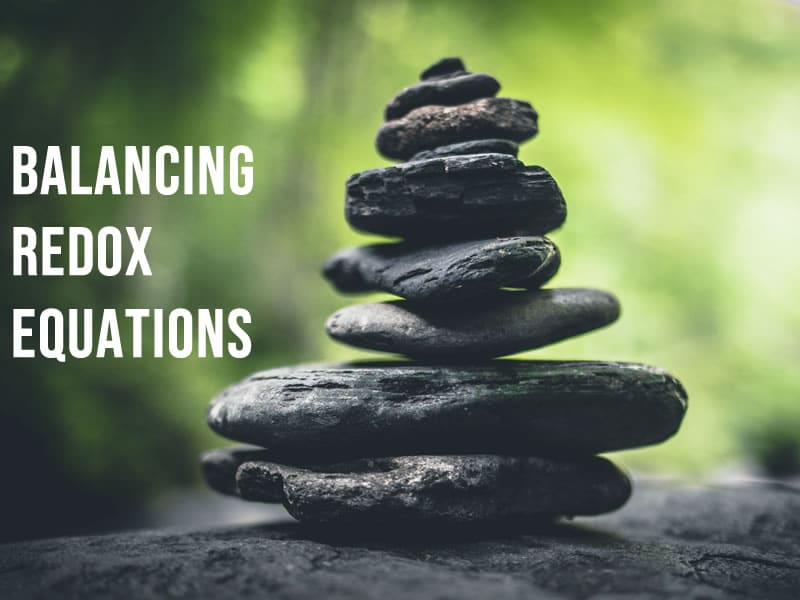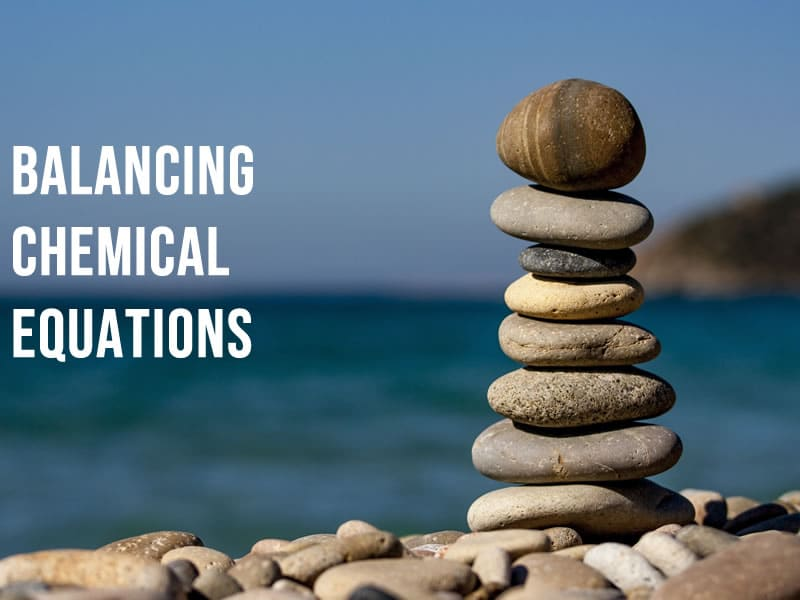# Chemical equations

•## Easy way to balance chemical equations for GCSE

Easy way to balance chemical equations for GCSE Balancing chemical equations A chemical equation balancing question is normally worth 1 or 2 marks, which means you have at most 2 minutes to solve [...]

2019-01-21T15:23:34+00:00January 18th, 2019|Categories: Essential Skills, Guides||0 Comments
•## Balancing chemical equations using algebra

Balancing chemical equations using the algebraic method In the last of my series on balancing chemical equations, we look at the algebraic method, which is useful for balancing the hardest equations Whilst [...]

2018-12-06T16:40:46+00:00December 3rd, 2018|Categories: Essential Skills, Guides||0 Comments
•## What are ionic equations and ionic half-equations?

Step-by-step guide to understanding and writing ionic equations Aimed at GCSE, A Level and IB students, this guide will help you master the difficult skills of writing half-equations and ionic half-equations [...]

2018-12-06T16:41:16+00:00November 17th, 2018|Categories: Essential Skills, Guides||2 Comments
•## Balancing redox reactions

Oxidation number method for balancing redox reactions The combination of redox and balancing chemical equations is enough to strike fear into the heart of any chemistry student. Read on to learn how to [...]

2019-01-08T14:35:38+00:00November 6th, 2018|Categories: Essential Skills, Guides||3 Comments
•## Balancing chemical equations

How to balance chemical equations Chemistry students often struggle with balancing chemical equations, but these tips will make this vital skill easier to master Is there an easy way to balance chemical [...]

2019-01-18T09:07:49+00:00October 31st, 2018|Categories: Essential Skills, Guides||3 Comments